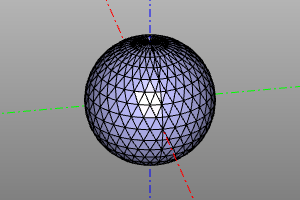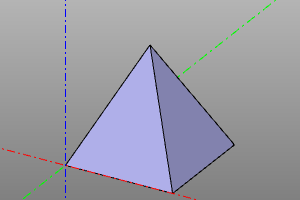Triangulation and mesh

EXPERIMENTAL

Functions for working with polygonal representation.

Triangulation

Creation of a polygonal mesh in the format (nodes, triangles), where pnts is an array of points, and triangles is an array of 3-tuples, indices of points. The deflection parameter is responsible for resolving the splitting.

Signature:

nodes, triangles = triangulate(shp, deflection)

Example:

m=sphere(10)
nodes, triangles = triangulate(m, 0.1)

print("count_of_nodes:", len(nodes))
print("count_of_triangles:", len(triangles))

print("first_five_nodes:", nodes[:5])
print("first_five_triangles:", triangles[:5])

#count_of_nodes: 699
#count_of_triangles: 1362
#first_five_nodes: [point3(0.000000,-0.000000,10.000000), point3(0.000000,-0.000000,10.000000), point3(0.000000,-0.000000,-10.000000), point3(1.950903,-0.000000,-9.807853), point3(3.826834,-0.000000,-9.238795)]
#first_five_triangles: [[237, 227, 200], [486, 482, 470], [237, 200, 211], [487, 472, 477], [238, 201, 212]]

Polyhedrone

A solid consisting of flat faces, specified by vertex points pnts and an array of tuples of indices of points defining the faces.

Signature:

polyhedron(pnts, faces, shell=False)

Example:

m=sphere(10)
nodes, triangles = triangulate(m, 0.1)
disp(polyhedron(nodes, triangles))Convex hull

Construction of the convex hull of a set of points. The scipy.spatial.ConvexHull procedure is used

convexhull_ computes an array of convex hull polygon point indices. convexhullshape builds a convex hull using the polyhedron procedure.

Options: incremental and qhulloptions_ are scipy.spatial.ConvexHull options (see scipy documentation). shell - create a shell instead of a body.

Signature:

convex_hull(pnts, incremental=False, qhull_options=None)
convex_hull_shape(pnts, shell=False, incremental=False, qhull_options=None)

Example:

pnts = points([
( 0,  0,  0),
(10,  0,  0),
(10, 10,  0),
( 0, 10,  0),
( 5,  5, 10),
])

print(convex_hull(pnts))
disp(convex_hull_shape(pnts))